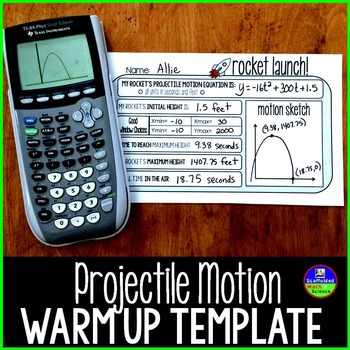# Quadratic Word Problems Projectile Motion Template9th - 11th
Subjects
Standards
Resource Type
Formats Included
• PDF
Pages
1 page

#### Also included in

1. This bundle contains all of the resources you will need to teach a summer school algebra class. Resources inside cover: solving equations, slope, linear equations, functions & domain and range, polynomials, systems of equations, factoring quadratics and the quadratic formula. For a bundle of eve
\$36.00
\$54.50
Save \$18.50
2. This bundle includes a variety of activities, resources and supports for your quadratics unit. Factoring quadratics, using algebra tiles, solving quadratic word problems, the Quadratic Formula and graphing quadratic functions. All resources in this bundle are included in this larger Algebra Activiti
\$40.00
\$59.50
Save \$19.50

### Description

This template helps structure the word problems that come up during your quadratics unit. Students use the template while finding the parts of parabolas and appropriate graphing calculator windows when graphing quadratics.

How I used the template:

I'd shine either an equation or a word problem on the board and give each student a template. Students would then write their equation on their template then find: initial height, a good graphing calculator window, time to max height, max height, and total time in air. They also sketch and label their graphs. There is a free set of PowerPoint slides with word problems linked inside.

You may also like:

Quadratic Word Problems Digital Math Escape Room

Total Pages
1 page
N/A
Teaching Duration
N/A
Report this Resource to TpT
Reported resources will be reviewed by our team. Report this resource to let us know if this resource violates TpT’s content guidelines.

### Standards

to see state-specific standards (only available in the US).
Use the process of factoring and completing the square in a quadratic function to show zeros, extreme values, and symmetry of the graph, and interpret these in terms of a context.
Graph linear and quadratic functions and show intercepts, maxima, and minima.
Graph functions expressed symbolically and show key features of the graph, by hand in simple cases and using technology for more complicated cases.
Create equations in two or more variables to represent relationships between quantities; graph equations on coordinate axes with labels and scales.